# greedy algorithms

mst   Prim   Kruskal   union-find   K correct   K complete   better UF

## mst

minimum spanning trees
• notes on msts, kruskal correctness, kruskal completeness

• definitions

• graph, path, connected, component, cycle, forest, tree, spanning, weighted graph, mst

• tree properties

• removing cycle edge does not change components

• tree with at least 2 nodes has at least 2 nodes with degree 1

• tree with n nodes has n-1 edges

• connected, n node, n-1 edges implies tree

• tree if and only if, between each node pair, unique path

• T spanning tree of G, e not in T, then

• T+e has cycle C

• for every c in C, T+e-c spanning tree

• if T mst, then w(e) ≥ w(c)

• X edge subset of mst T, S node subset of V such that no x crosses cut (S,V-X), e lightest edge across cut, then X+e edge subset of mst T’

• sedgewick/wayne slides

## Prim's mst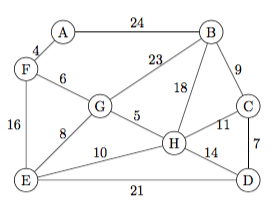trace Prim from E
```            A    B    C    D    E    F    G    H
tree                            *
E update                  21 E  *   16 E  8 E 10 E
GE 8                            *         *
G update        23 G      21 E  *    6 G  *    5 G
HG 5                            *         *    *
H update        18 H 11 H 14 H  *    6 G  *    *
FG 6                            *    *    *    *
F update    4 F 18 H 11 H 14 H  *    *    *    *
AF 4        *                   *    *    *    *
A update    *   18 H 11 H 14 H  *    *    *    *
CH 11       *         *         *    *    *    *
C update    *    9 C  *    7 C  *    *    *    *
DC 7        *         *    *    *    *    *    *
D update    *    9 C  *    *    *    *    *    *
BC 9        *    *    *    *    *    *    *    *
```
simple Prim mst
```def prim(G,source):
cost, parent, fringe = {}, {}, []
infin = weighted.infinity(G)
for v in G:
cost[v], parent[v] = infin, -1
fringe.append(v)
cost[source],parent[source] = 0, source

sum = 0
print source, 'start'
while len(fringe)>0:
u = fringe.pop(weighted.indexOfMin(fringe,cost))
sum += cost[u]
if u != source: print u,parent[u],cost[u]
if cost[u] == infin:  doneEarly = True
for (v,costuv) in G[u]:
if (v in fringe) and (cost[v] > costuv):
cost[v] = costuv
parent[v] = u
print 'mst weight', sum

prim(weighted.G4,'A')
```

## Kruskal's msttrace Kruskal
```AF 4
GH 5
FG 6
CD 7
EG 8
BC 9
reject EH
CH 11
reject DH
reject EF
reject BH
reject DE
reject BG
reject AB
```
implementing Kruskal
• what component are you in?

• what component are you in?

• merge components containing x,y

• leads to a union-find data structure

• simplest implementation

• component: tree, with parent pointers

• find(a): return root of tree containing a

• union(a,b): parent[root(a)] = root(b)

## simple union find data structure

```def myfind(v,P): # simple find
while P[v] != v:
v = P[v]
return v

def myunion(x,y,P): # simple union
rootx, rooty = myfind(x,P), myfind(y,P)
P[rootx] = rooty
```
simple Kruskal
```import weighted, UF
# convert from weighted adjacency list
#         to tuple list:   (v,w,wt_vw)
def createEdgeList(G):
L = []
for v in G:
for (w,wt) in G[v]:
if ord(v)<ord(w): L.append((v,w,wt))
return L

# extract tuple with min wt
def extractmin(L):
ndxMin, valMin = 0, L
for j in range(1,len(L)):
t = L[j]
if t < valMin:
ndxMin, valMin = j, t
return L.pop(ndxMin)

def kruskalDemo(G):
L = createEdgeList(G)
parent = {}
for v in G: parent[v] = v

while len(L) > 0:
t = extractmin(L)
a, b = t, t
ra, rb = UF.myfind(a,parent), UF.myfind(b,parent)
print a, ra, b, rb,
if ra != rb:
UF.myunion(ra,rb,parent)
else:
print 'reject  ',a,b

kruskalDemo(weighted.G4)
```
trace: Kruskal with union/find data structure
```a r b s    r,s: roots of UF comp'ts with a,b
parent
A B C D E F G H
A A F F add A F 4    F B C D E F G H
G G H H add G H 5    F B C D E F H H
F F G H add F G 6    F B C D E H H H
C C D D add C D 7    F B D D E H H H
E E G H add E G 8    F B D D H H H H
B B C D add B C 9    F D D D H H H H
E H H H reject   E H
C D H H add C H 11   F D D H H H H H
stop: n-1 edges
final UF structure:              H
F   G   E   D
A          C B
```

## Kruskal mst correctness

• Claim: tree returned by Kruskal is mst.

• Proof (sketch)

• at each step, set of picked edges is acyclic, so a forest.

• number of components of n-node m-edge acyclic forest is n-m (exercise: PBI)

• so, when number picked edges < n-1, still ≥2 components. since input graph connected, must be an edge in input graph with ends different forest components. so, K's alg can pick some edge.

• so K's alg picks n-1 edges, leaving acyclic graph, n nodes, 1 component, so spanning acyclic component, so spanning tree.

• Claim: the tree returned is an MST.

• Proof (sketch):

• Let T be the tree returned by Kruskal's algorithm. Let T* be an MST. If T=T* we are done, so assume T ≠ T*.

• Label the edges of T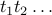in the order they are selected by Kruskal's algorithm, i.e. non-decreasing weight.

• Let k be the smallest index such that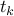is not in T*.

• Notice that T*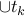has a cycle C containing, and that removing any edge of C leaves a spanning tree

• why? because the resulting graph is connected, and has n-1 edges

• Notice that C contains at least one edge t* that is not in T

• why? because T is a tree, so acyclic

• Notice that the weight of every such t* is at least the weight of• why? because t* does not form a cycle with edges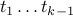, which are all in T*, and yet Krusal pickedinstead of t*

• Notice that the weight of every such t* is at most the weight of• why? because T' = T* -t* +is a spanning tree, with weight wt(T*) - wt(t*) + wt(), so if wt(t*) > wt() then wt(T') < wt(T*), contradiction since T* is an MST

• so, for every such t*, wt(t*) = wt(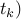• pick any t*, and let T' = T* -t* +• notice T' is an MST, with one more edge of T than T*

• so repeating the above process as necessary, we eventually find an MST that contains all the edges of T, i.e. that equals T.

## Kruskal mst completeness

every mst is kruskal
• recall: in each step of K's algm, any min-cost candidate edge can be added to the tree-so-far

• call an mst kruskal if it is found by some execution of K's algm

• claim: every mst is Kruskal

proof: by induction, contradiction form: consider an arbitrary mst T of a graph G. among all possible Kruskal executions on G, consider the one that yields the Kruskal mst K that maximizes the initial sequence of edges that are in T. say the sequence of edges of this execution is S=( k1, ..., kn-1) . so these edges form the mst K.

let q be smallest index such that kq is not in T.

T+kq has a unique cycle C. The edges of A={ k1, ..., kq } are picked by Kruskal, so acyclic, so C includes some edge not in A, say c.

kq is a max weight edge on C. But Kruskal picks kq before c, so kq weighs no more than c. So kq weighs the same as c.

So Kruskal could have picked c instead of kq. So some execution of Kruskal starts by selecting the edge sequence ( k1, ..., kq ) , contradicting our choice of K.

## better UF

Kruskal UF structure: can we do beter?
```what is total cost of these operations?

union(A B)  union(B C)  union(C D) ... union(Y Z)
find(A) find(B) find(A) find(D) ... find(A) find(Z)

motivation: keep UF trees shorter

two options: union-by-rank, or find-grandparent-compress
```
better UF
```def findGP(x, parents): # find, gp compress
px = parents[x]
if x==px: return x
gx = parents[px] # grandparent
while px != gx:
parents[x] = gx
x = px ; px = gx ; gx = parents[gx]
return px

def unionBR(v,w,P,R): # union by rank
rv,rw = myfind(v),myfind(w)
if   R[rv] < R[rw]:
P[rv] = rw
elif R[rv] > R[rw]:
P[rw] = rv
else:
P[rv] = rw
R[rw] += 1
```
min cuts: randomized algorithm
• min cut of a graph: cut (S,V-S) that minimizes number of cross edges

• brute force algorithm: consider all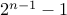possible cuts

• Karger's randomized algm:

• single iteration: Kruskal (except select edges at random), stop after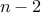edges

• with prob. at least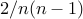, cut is min

• perform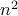iterations, take smallest cut, with high prob is min

Karger: prob cut min at least 2/n(n-1)
• consider min cut, say size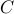• on first step,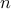components

• each component incident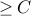edges

• so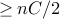edges

• Prob(picking edge from)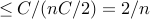• when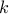components

• each component incidentedges

• so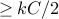edges

• if so far have missed,
Prob(picking edge from)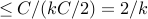• prob(avoidingeach time)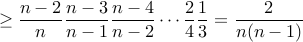Karger: an example
• consider the graph with vertices A to F and edges AB AC BC DE DF EF plus AD

• uniqe min cut {A B C} {D E F} has size 1 (edge AD)

• probability that Karger's algorithm finds this cut?

• to find this cut, must pick 2 edges from each of 2 triangles

• prob that first edge from a triangle: 6/7

• prob that second edge from a triangle: 5/6

• two cases:

• i) prob 2/5: one triangle has 1 edge remaining, the other 3

• ii) prob 3/5: each triangle has 2 edges remaining

• prob third edge from a triangle: i) 3/4 (one of 5 remaining edges would create a cycle if picked) ii) 4/5

• prob fourth edge from a triangle: 2/3 (one of 4 remaining edges would create a cycle if picked)

• total prob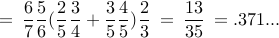• general bound ? 6-vertex graph, prob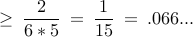randomized min cut
```import random

def myfind(x, parents):
if x==parents[x]: return x
return myfind(parents[x],parents)

def myunion(x,y,parents):
parents[x] = y

def nodeedgecount(G):
n,m = 0,0
for v in G:
n+= 1
for w in G[v]:
if v < w: m+= 1
return n,m

def edgelist(G):
L = []
for v in sorted(G):
for w in G[v]:
if v < w: L.append((v,w))
return L

def cutedges(E,P):
edgeset = []
for e in E:
if myfind(e,P) != myfind(e,P):
edgeset.append(e)
return edgeset

def makeparents(G):
p = []
for v in sorted(G):
p.append(v)
return p

def randomcut(G,n,E):
P = makeparents(G)
for e in E:
x = myfind(e,P)
y = myfind(e,P)
if x != y:
myunion(x,y,P)
if edgesadded == n-2:
return cutedges(E,P)

def showgoodcuts(G,t):
# iterate t times; show cut every time it improves
n,m = nodeedgecount(G)
E = edgelist(G)
bestcutsize = m+1 # initialize with impossible value
for j in range(t):
random.shuffle(E)
C = randomcut(G,n,E)
if len(C) < bestcutsize:
bestcut = C
bestcutsize = len(bestcut)
print 'iteration',j,bestcut

G = {0:[1,2,3], 1:[0,2,3], 2:[0,1,3,4], 3:[0,1,2,5],
4:[2,5,6,7], 5:[3,4,6,7], 6:[4,5,7], 7:[4,5,6] }

showgoodcuts(G,10)
```
Huffman encoding